The circumspect engineer, given a problem to solve, inevitably faces a wide range of plausible alternative design choices. Typically, alternative paths to solving a problem differ in various cost and performance parameters, including
• Hardware cost measures: circuit size, transistor count, etc.
• Performance measures: Latency, throughput, clock speed.
• Energy cost measures: average/maximum power dissipation, power supply and cooling requirements .
• Engineering cost measures: design ease, simplicity, ease of maintenance/modification/extension.
Engineering choices typically involve optimizing certain of these parameters, often at the expense of others. In this chapter we explore several examples of such tradeoffs.
Optimizing Power

## 12.1. Optimizing Power

We noted in Section 6.5 that the primary cause of power dissipation within CMOS logic circuitry is ohmic losses in the flow of current into and out of signal nodes during logic transitions. As observed in that section, the power dissipated is proportional to the frequency of transitions, which, in typical circuitry, is proportional to the clock frequency.

In many situations we can improve the average (or maximum) energy dissipation by choosing a design that minimizes the number of logic transitions per second. A simple way to achieve that goal is by lowering the clock frequency, usually at a proportional loss of processing performance; indeed, some modern computers have low-power operating modes which simply slow the clock to a fraction of its maximal rate.

Eliminating Unnecessary Transitions

### 12.1.1. Eliminating Unnecessary Transitions

Can we lower the rate of transitions without commensurate performance degradation? Often the answer is yes. Consider, for example, the design of an Arithmetic Logic Unit (ALU), a high-level combinational building block you will be familiar with from the laboratory. An ALU is a computational Swiss army knife, performing any of a repertoire of operations on its input data based on a multi-bit function code (ALUFN) passed as a control input.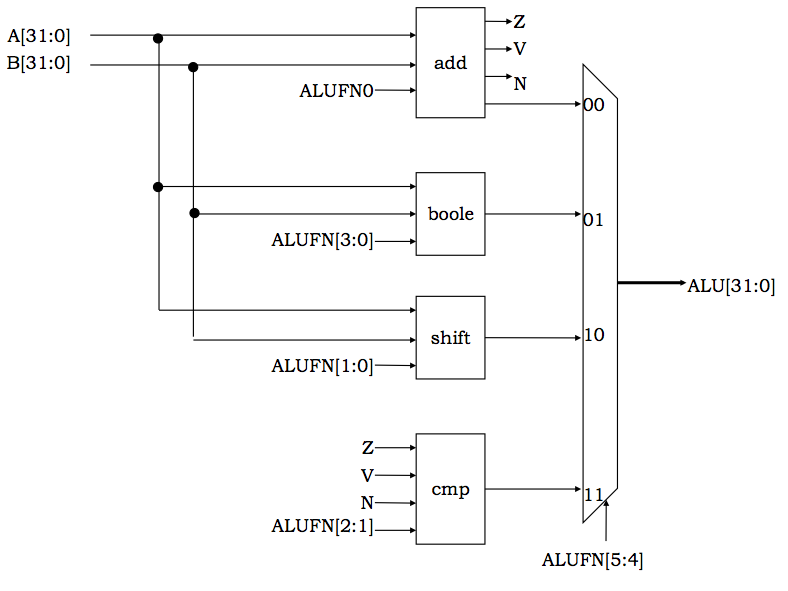Typical ALU Block Diagram
The diagram to the right shows a typical top-level design for a combinational ALU: there are four subsystems responsible for different classes of operations (addition, Boolean, etc) each of which operates simultaneously on the input data. A final four-way multiplexor selects the computed result actually requested by the ALUFN code, discarding the values needlessly computed by the unselected subsystems.

Assuming the requested operation is the exclusive or $A \oplus B$ of two 32-bit input operands $A$ and $B$, the 32-bit sum $A + B$ by the adder unit has simply wasted whatever power is taken for its computation. The redundant computation will automatically take place in our combinational circuit as soon as the $A$ and $B$ inputs take on new values, with many consequent transitions and power dissipation throughout the adder circuitry.Power-saving ALU Design
We can eliminate these redundant transitions simply by not allowing the inputs to the adder to change unless an add operation has actually been selected. One way to accomplish this is shown to the left. In this approach, latches are placed in the data paths to each subsystem, and are gated by the particular combination of ALUFN bits that selects a computation by that subsystem. Using this scheme, the $A$ and $B$ inputs to unselected subsystems will be frozen, forcing internal logic nodes of these circuits to retain constant values and thereby dissipate very little power.
Optimizing Latency

## 12.2. Optimizing Latency

We can often use the mathematical properties of operations performed by circuit elements to rearrange their interconnection in a way that performs equivalent operations at lower latency. A common class of such rearrangements is the substitution of a tree for a linear chain of components that perform an associative binary operation, mentioned in Section 7.7.2.
Parallel Prefix

### 12.2.1. Parallel Prefix

We can generalize this approach to other binary operations. Suppose $\circledast$ is an associative binary operator, meaning that $a \circledast (b \circledast c) = (a \circledast b) \circledast c$. We can generalize this operator to $k$ operands $x_0, x_1, ... x_{n-1}$ by iteratively applying the binary $\circledast$ operator to successive prefixes of the operand sequence, computing each intermediate $x_j \circledast ... \circledast x_0$ by the recurrence $x_j \circledast (x_{j-1} \circledast ... \circledast x_0)$, generating values for each successive prefix of the argument sequence.

This imposes a strict ordering on the component applications of the binary $\circledast$ operator; it requires that the result of applying $\circledast$ to the first $j-1$ arguments be completed before it is applied to $x_j$. In a sequential circuit implementation, the $k$-operand function would take $\theta(k)$ execution time, sufficient for a sequence of $k$ binary operations. A corresponding combinational circuit would have an input-output path going through all $k-1$ components, again implying a $\theta(k)$ latency.

The associativity of $\circledast$, however, affords some flexibility in the order of the component computations. We can use the fact that $$x_0 \circledast (x_1 \circledast (x_2 \circledast x_3)) = (x_0 \circledast x_1) \circledast (x_2 \circledast x_3)$$ to process pairs of arguments in parallel, reducing the number of operands to be combined by a factor of two in the time taken by one binary operation. Repeated application of this step -- halving the number of operands in a single constant-time step -- reduces the asymptotic latency from $\theta(k)$ to $\theta(log(k))$, a dramatic improvement. In a combinational implementation, it results in a tree structure like that of Section 7.7.2.

The key to this (and other) latency reduction techniques is to identify a critical sequence of steps that constrains the total execution time, and break that sequence into smaller subsequences that can be performed concurrently.

Returning to the problem of binary addition, we have observed that the critical sequence through the ripple-carry adder of Section 11.2 is the combinational path that propagates carry information through each full adder from low-order to high-order bit positions. We might (and generally do) aspire to minimize the delay along this path by careful design of our full adder component to produce the output carry as fast as possible; however, this optimization gives at best a constant factor improvement to our $\theta(N)$-bit latency.

More dramatic improvements require that the carry chain somehow be broken into pieces that can be dealt with in parallel. Suppose we need a 32-bit adder, but are unhappy with the latency of a 32-bit ripple-carry adder. We might observe that the latency of a 16-bit adder is roughly half that number, owing to its shorter carry chain; thus we could double the speed of our 32-bit adder by breaking it into two 16-bit adders dealing in parallel with the low- and high-order halves of our addends. The problem is that the carry input to the high-order 16-bit adder is unknown until the low-order adder has completed its operation.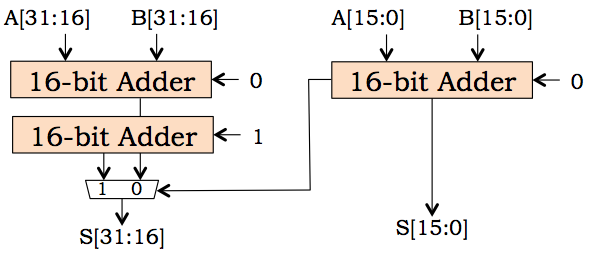To accommodate this uncertainty, we simply replicate two instances of the high-order 16-bit adder, as shown to the right. We supply one of the high-order adders with $0$ as the carry input, the other with $1$; this guarantees that the sum bits coming from one of these high-order adders will be correct. We of course can't know which high-order sums to select, until the carry output is produced by the low-order add. To this end, we use a multiplexor to select between the two high-order sums depending on the low-order carry output.

The latency of this carry-select adder is that of the 16-bit adder plus the delay of the multiplexor, nearly a factor of two saving. It comes at a hardware cost of over 50%, however, owing to the redundant high-order adder. Of course the number of bits in the high- and low-order portions need not be equal; often the low-order portion has fewer bits, allowing time for its carry output to propagate through the multiplexors selecting the high-order outputs.

An $N$-bit carry-select adder may be hierarchical -- that is, each of the three component adders may themselves be carry-select adders. If we make a deep hierarchy of carry-select adders in this fashion, we can make a $2 \cdot n$-bit adder whose latency is only a mux delay longer than its three component $n$-bit adders, doubling $n$ at a fixed cost at each level. The result is a family of hierarchical $n$-bit carry-select adders whose latency grows as $\theta(\log(n))$.

It is tempting to try rearranging the full adders of a ripple-carry adder in a tree, in order to get the $\theta(log n)$ latency available to $n$-bit associative logical operations like AND and XOR. Of course, the functions performed by the full adders cannot be arbitrarily regrouped, since the full adder at each bit position takes as input a carry from the next lower-order position, and supplies a carry to the adjacent higher-order full adder. This would seem to rule out performing the function of the higher-order FAs simultaneously with that of lower-order ones.

Clever reformulation of the building blocks used for our adders, however, can mitigate this constraint. The key to our new approach is to eliminate carry out signals, which necessarily depend on carry inputs (leading to the $\theta(n)$ carry chain), replacing them with alternative information that can be used to deduce higher-order carry inputs.

We'd like the new outputs to have two key properties:

• They can be generated from the $A_i$ and $B_i$ inputs available at each bit position from the start of the operation, not depending on outputs computed from other bit positions;
• Information from two adjacent sequences of bit positions can be aggregated to characterize the carry behavior of their concatenation.

Consider the carry output from a $k$-bit ripple-carry adder. Given only the $k$-bit $A$ and $B$ data inputs, what can we say about the carry output it would produce? We can identify three cases:

• Generate: Given these $A$ and $B$ values, $C_{out} = 1$ always.
• Kill: Given these $A$ and $B$ values, $C_{out} = 0$ always.
• Propagate: Given these $A$ and $B$ values, $C_{out} = C_{in}$, where $C_{in}$ is the carry input to the low-order bit of the adder.
These three cases characterize the carry output independently of the carry input (in the sense that the characterization is valid whatever the carry input turns out to be). This allows it to be determined independently of the carry input -- i.e., in parallel for various bit positions within the adder.

Lets consider a family of $k$-bit adders that replace carry output signals with outputs that encode these three classes of carry behavior. Given 3 cases, at least 2 signals are required. We choose the following two:

• G (generate): $1$ if the carry is $1$;
• P (propagate): $1$ if the carry is $C_{in}$.
If neither G or P is asserted, then the carry is $0$ independently of $C_{in}$.

Given $G$ and $P$ signals and the input carry $C_{in}$, the carry output can be computed by $C_{out} = G + P \cdot C_{in}$.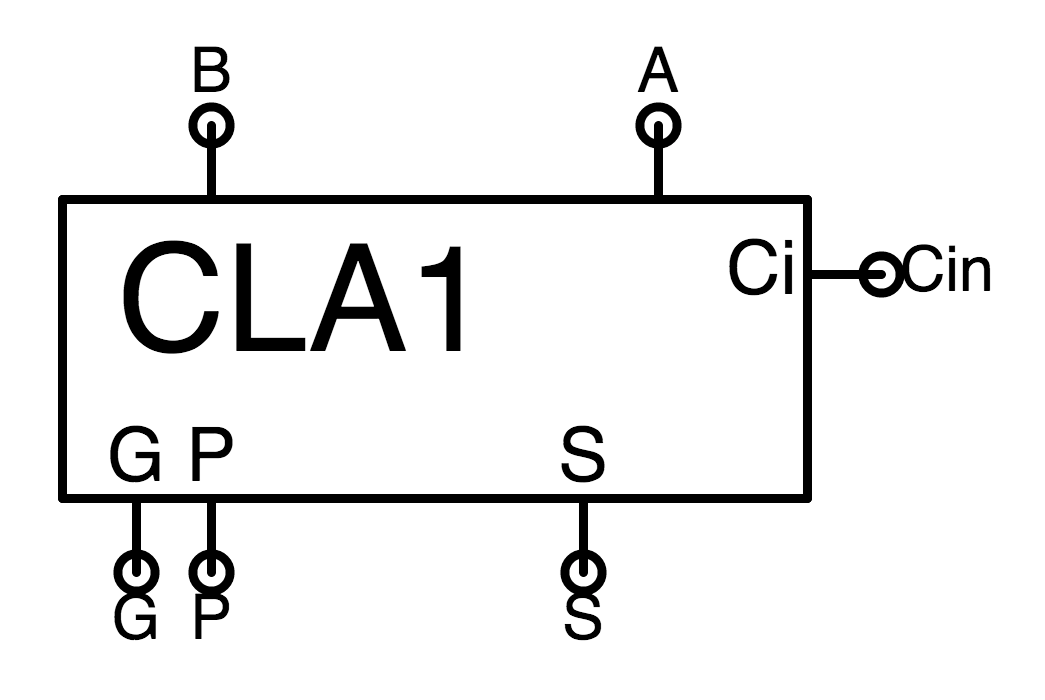1-bit CLA
The full adder of our ripple-carry implementations can be replaced by a $1$-bit carry lookahead adder. whose icon is shown to the left.1-bit CLA logic
Its sum output is $A \oplus B \oplus C_{in}$ as in the full adder, but $P = A \oplus B$ and $G = A \cdot B$ as shown to the right; this reflects the observation that an output carry is propagated if either $A$ or $B$ is one, and $generated$ if they are both one.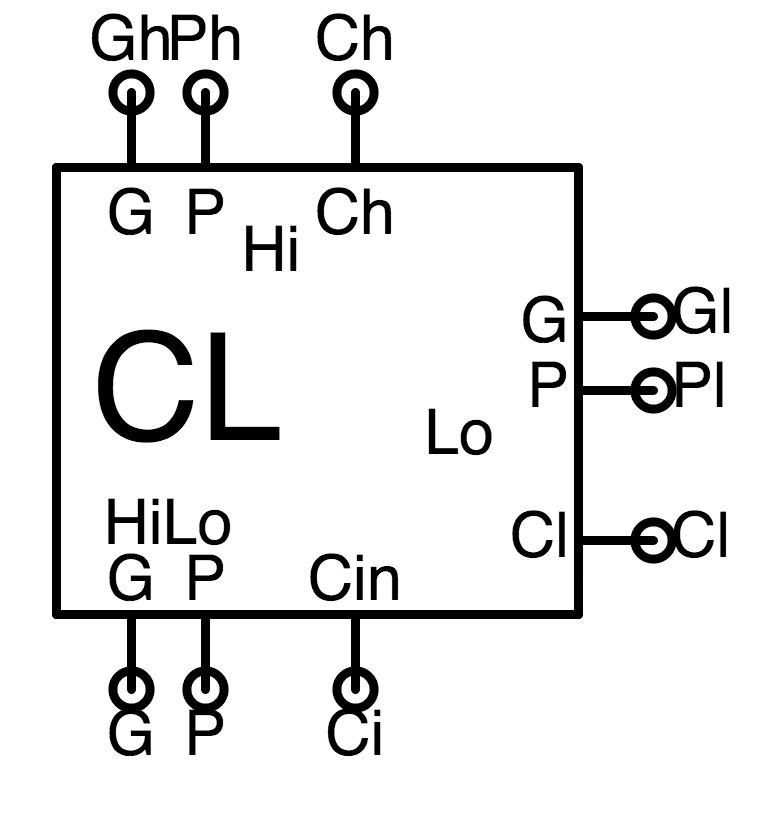Next we turn to the problem of cascading two small (say, $n$-bit) carry lookahead adders to make a bigger ($2 \cdot n$-bit) carry lookahead adder. To facilitate cascading, we define a specialized commponent, called CL for carry lookahead.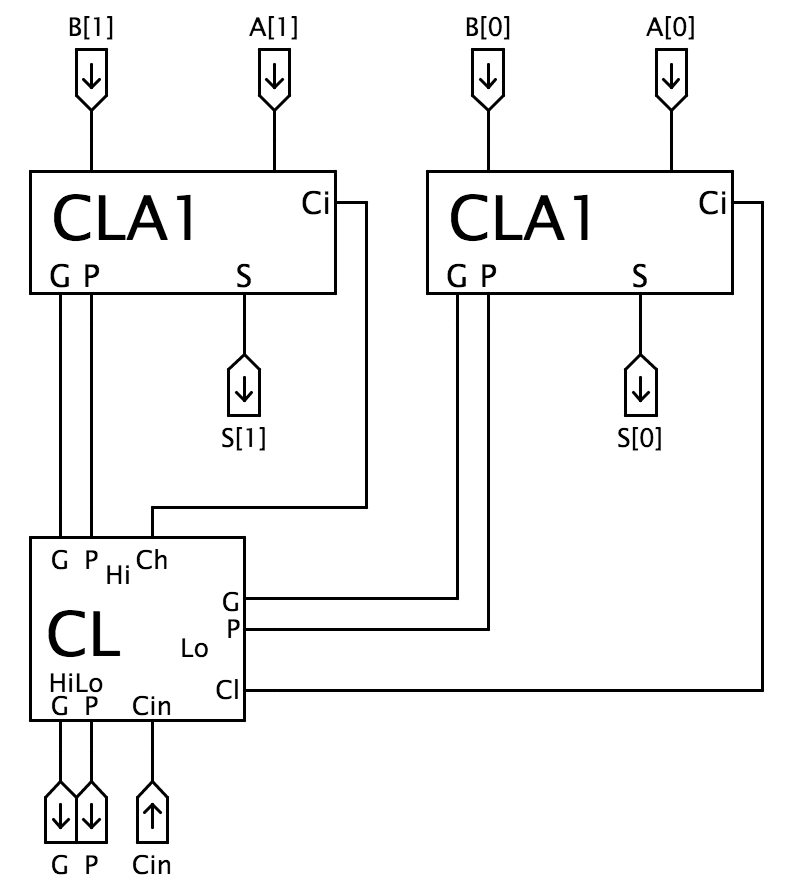2-bit CLA circuit
Each CL instance combines $P$, $G$, and $C_{in}$ signals of two carry-lookahead adders (say, $k$-bit and $j$-bits, respectively) to make them appear as a single, wider $k+j$-bit carry lookahead adder. It takes as input the carry input $C_{in}$ to the combined adder, and produces as output the $P$ and $G$ signals for the combination. The diagram on the left shows its use to combine two 1-bit carry lookahead adders into the 2-bit adder whose icon is nearly identical to that of the CLA1.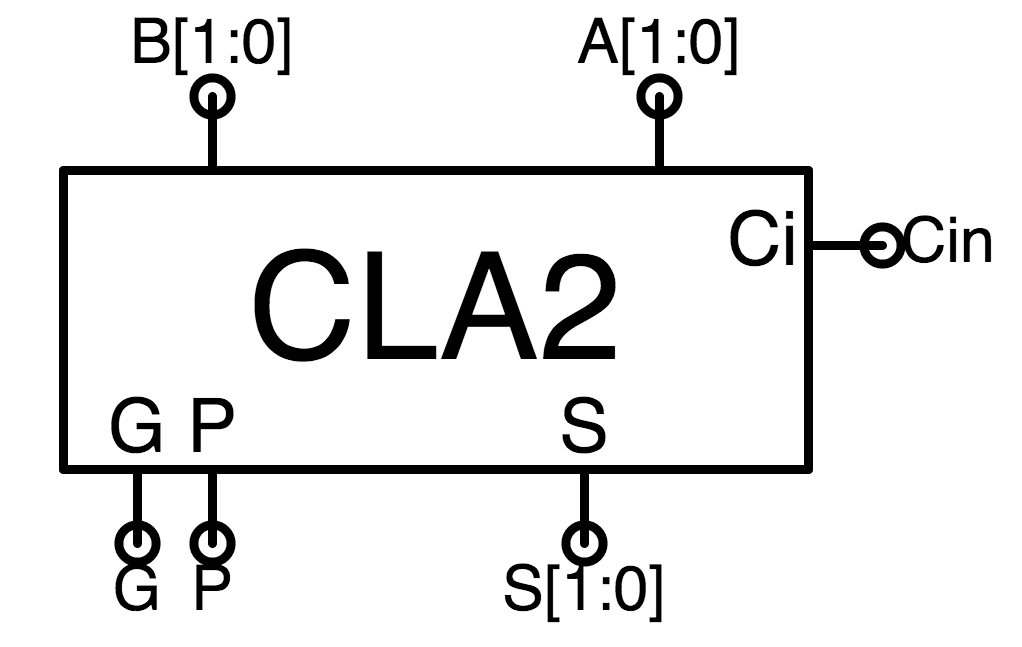2-bit CLA
Note that the 2-bit carry lookahead adder follows the same terminal pattern as the 1-bit version, differing only in the number of bits in the data signals.

We can combine two carry-lookahead adders of arbitrary sizes using a CL module. We allocate one component adder to the high-order portion of the sum and the other to the low-order, routing their $P$, $G$, and $C_{in}$ signals to the CL module. The CL module performs two separable functions:

•CL GP logic
It combines $G_h, P_h$ and $G_l, P_l$ signals from the high- and low-order component adders to generate appropriate $P$ and $G$ signals for their combination. The combined $P = P_h \cdot P_l$ and $G = G_h + P_h \cdot G_l$ are generated by the logic shown to the right.
• It generates carry input signals $C_h$ and $C_l$ for the component adders. The carry input to the low-order component is just the carry input to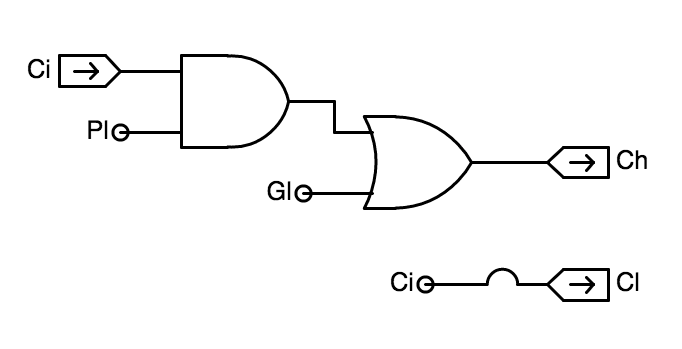CL carry logic
the combined adder; it is the $C_{in}$ input to the CL component. The carry input to the high-order component is computed using the $P$ and $G$ inputs computed in the step above, using the pair of gates shown to the right.
The observant reader might notice that the 2-bit CLA circuit shown above appears to contain combinational cycles: it takes $P$ and $G$ signals from the component adders, and produces $C_{in}$ signals that are routed back to each adder. However, the two combinational functions performed by our CL module are entirely independent of one another; they are simply combined, for convenience, in a single module. Separating the CL module into independent combinational devices would allow us to redraw the diagram in acyclic form, verifying that it is indeed a valid combinational circuit.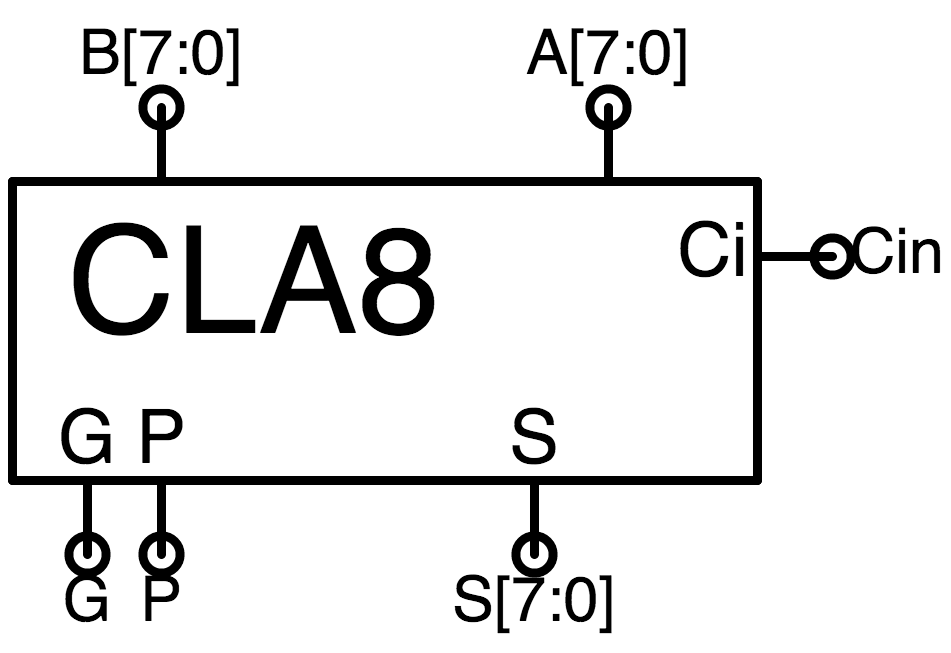8-bit CLA
We can combine two CLA2 modules (using another CL component) to make a 4-bit CLA4, two CLA4s to make an 8-bit CLA8, etc. Moreover, we can use a CL module to combine, say, 16-bit and 4-bit carry lookahead adders to make a 20-bit adder; the CL device operates independently of the number of bits in each component adder.

Each time we use a single CL module to combine two adders, we are in effect adding a layer to a tree of logic. If we expand the diagram of an 8-bit adder made this way, we get the circuit shown below: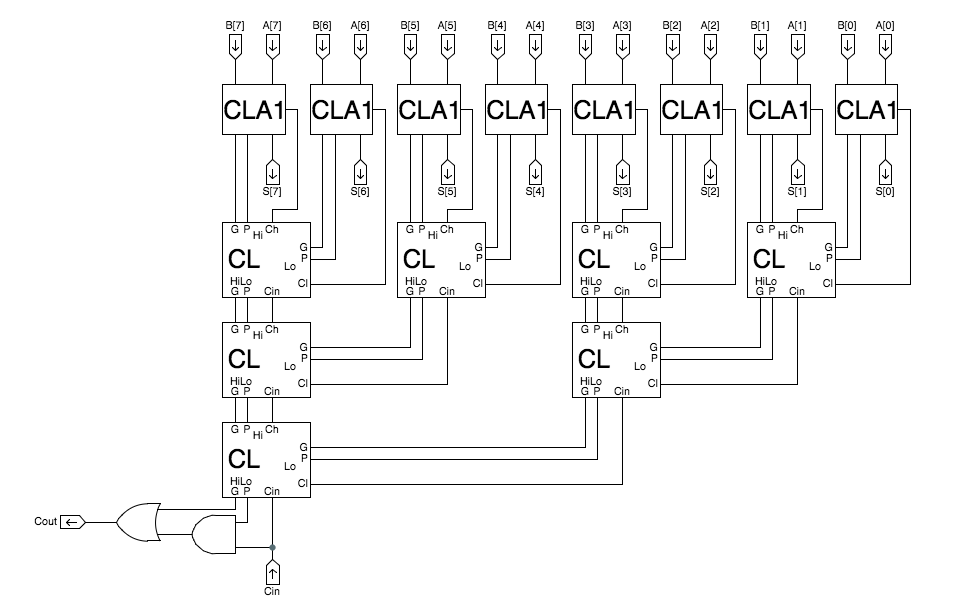8-bit CLA, expanded
Observe that we have added two gates to compute a carry output, $C_{out}$, from the $P$ and $G$ outputs of the bottommost CL block. This allows our carry lookahead adder to replace slower ripple-carry adders that produce carry outputs.

Note that the "leaves" of the tree -- the 1-bit CLA1 components -- get all of their $A$ and $B$ data bits simultaneously at the start of the ADD operation, independently of the number of bits. They each produce $P$ and $G$ signals independently of their late-arriving $C_{in}$ inputs; these $P$ and $G$ values propagate to the root CL element in the tree, which computes $C_{in}$ values that propagate through the tree back to the leaves.

Using this construction, we can build a $2^k$-bit adder with a tree of depth $k$. The worst-case propagation path through this circuit is the $P$, $G$ values to the root CL followed by carry propagation back to the leaves -- a path whose propagtion delay is proportional to the tree depth $k$ rather than the bit-width $2^k$. The latency of a $2^k$-bit adder built this way is thus $\theta(k)$, whence the latency of an $N$-bit adder is $\theta(log N)$.

Optimizing Throughput

## 12.3. Optimizing Throughput

The most obvious way to improve the throughput of a device that computes $P(X)$ from $X$ is brute-force replication: if the throughput of one device is $T_P$ computations/second, then $N$ copies of the device working in parallel can perform $N \cdot T_P$ computations/second. In Section 11.5 we introduced the notion of pipelining, which often affords cheaper throughput improvements by adding registers rather than replicating the logic that performs the actual complication.

These two techniques can often be combined to optimize throughput at modest cost. However, computation-specific engineering choices can dramatically impact their effectiveness and the performance of the resulting design.

N-bit Binary Multiplier

### 12.3.1. N-bit Binary Multiplier

Consider, for example, the multiplication of two $N$-bit unsigned binary numbers $A_{N-1:0}$ and $B_{N-1:0}$ to produce a $2 \cdot N$-bit unsigned binary product $P_{2N-1:0}$. We may view this operation as the sum of $N$ partial products, each of the form $B_i \cdot A_{N-1:0} \cdot 2^i$ for $0 \leq i \lt N$.

Observe that each partial product can be formed by $N$ AND gates, multiplying the bits of $A$ by a selected $B_i$. It remains to sum the $N$ partial products, which we can do using an array of full adders -- much like our $N$-bit by $M$-word adder of Section 11.4. To streamline our design, we devise a multipler "slice" component containing $N$ full adders with an AND gate on one sum input, and replicate this slice component for each of the $N$ partial products. Our resulting design has the following structure: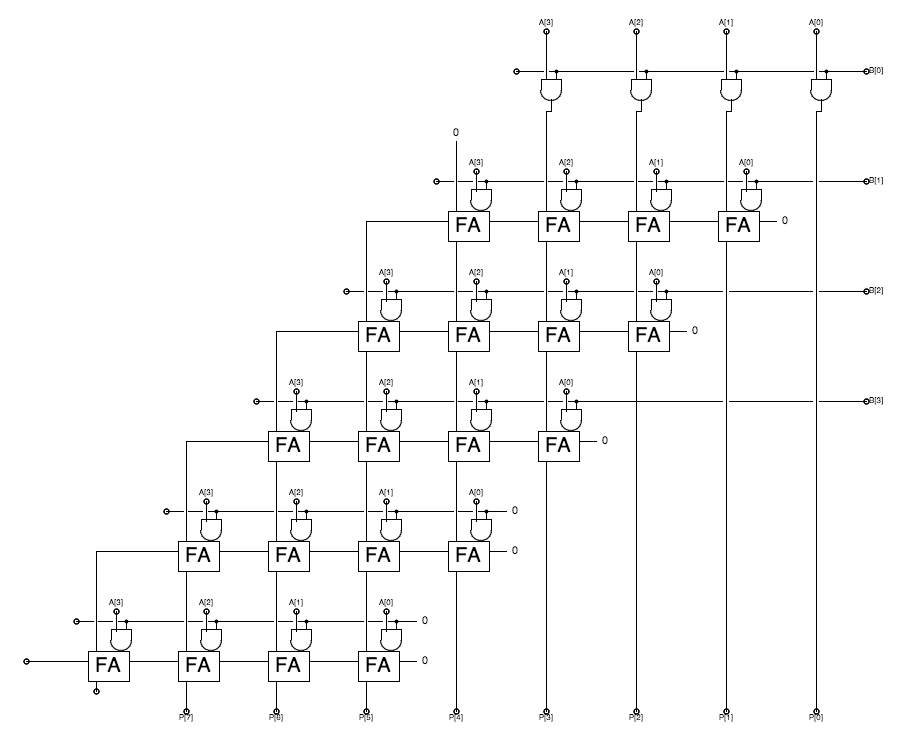4-bit Multiplier
Note the two-dimensional layout of the diagram. Each row or "slice" has four locations, forming the 4-bit partial product $A \cdot B_i$ for consecutive bits $B_i$ of $B$ in consecutive rows. Each row is offset to properly account for the weight of its contribution to the final product, and the rightmost bit of each partial product becomes part of the product formed at the bottom terminals.

The topmost slice contains only AND gates, as it has no incoming value from rows above to add to the partial product it generates. Subsequent rows have a full adder at each bit position, to combine the incoming sum from above with the partial product generated by the AND gates. Each row effectively contains a ripple-carry adder as well as the AND gates that form the partial product.

Although there are only 4 4-bit partial products, we use additional slices to propagate carries and form the full $2\cdot N$ bits of the final product. The full adders in these slices have unused data inputs connected to logical 0 (ground), representing that $B_i = 0$ for $i \geq 4$. As a result, these additional bit positions don't require the logic of a full adder, and could be simplified considerably; similarly, the rightmost full adder in each slice might be optimized in view of its carry input tied to 0.

While such optimizations are straightforward, we will not pursue them here. Noting that these optimizations may reduce constant factors impacting the cost and performance parameters of our multiplier, they do not effect its asymptotic cost or performance. The above 4-bit multipler generalizes to an $N$ bit combinational multipler, whose two-dimensional structure reflects an asymptotic hardware cost of A$\theta(N^2)$. Like the $N$-bit by $M$-word adder of , the signal flow through the array of full adders is top-to-bottom and right-to-left, leading to a longest-path propagation delay that grows as the sum of the array's width and height -- each $\theta(N)$. Thus the latency of our combinational multiplier is $\theta(N+N) = \theta(N)$, and its throughput is $\theta(1/N)$.

Pipelined Multiplier

#### 12.3.1.1. Pipelined Multiplier

If we have a large number of independent multiplications to perform, it seems logical to pipeline our $n$-bit combinational multiplier to improve its $\theta(1/N)$ throughput. Quick inspection of the $4$-bit multiplier diagram above suggests an obvious partitioning of the combinational circuit into pipeline stages: each horizontal slice, that forms a 4-bit partial product and adds it to the sum coming from slices above, might constitute a pipeline stage. Indeed, we could pipeline our multiplier by drawing stage boundaries between horizontal slices, and inserting registers wherever a data path intersects a stage boundary.

Unfortunately, such a pipelining approach would not result in improved performance. Since each multiplier slice effectively contains an $N$-bit ripple-carry adder, it requires $\theta(N)$ time for the carry to propagate from right to left through all $\theta(N)$ bits. The maximal clock period is thus $\theta(N)$, and the throughput of our naively pipelined multiplier remains at $\theta(1/N)$. Since there are $\theta(N)$ stages, however, the latency has now been degraded from $\theta(N)$ to $\theta(N^2)$.

To materially improve performance, we must find a way to break long ($\theta(N)$) paths within each pipeline stage. The problem with our naive pipeline is that our horizontal pipeline boundaries failed to break up the horizontal carry chains within each slice. We might address this problem by making either the pipeline stage boundaries or the carry chains less horizontal, so that they intersect.

The latter approach is deceptively simple: rather than routing the carry output from each full adder to the full adder on its immediate left, we route the carry to the corresponding full adder in the slice below.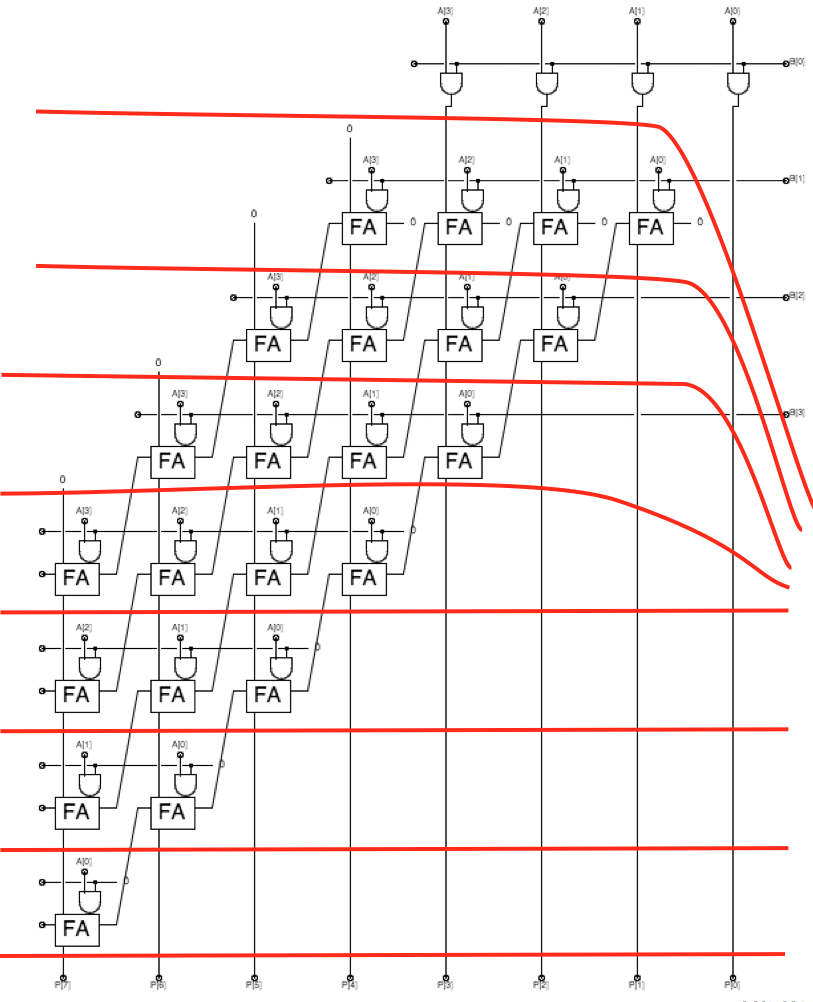4-bit Multiplier, pipelined
With this minor change, horizontal pipeline stage boundaries intersect both the vertical path of the sum formation and the diagonal path of carry propagation. The worst-case propagation delay through each slice is constant -- $\theta(1)$ -- rather than $\theta(N)$, allowing a constant clock frequency independently of the number $N$ of operand bits.

Making this design work requires careful attention to a number of details we gloss over here. Among these is the need for pipeline registers at points where data lines are implicit in the diagram -- for example, those carrying the 4-bit $A$ operand to the various pipeline stages.

Sequential Multiplier

#### 12.3.1.2. Sequential Multiplier

The $N$-bit pipelined multipler just sketched can be built using $\theta(N)$ idential "slices", each a module containing $N$ each AND gates and full adders; we note that the topmost slice in our diagram omits the full adders, optimizing for the case when the sum coming from the slice above is zero. In pursuit of high throughput, at any instant each of the identical slices of our pipelined problem will be working on a different multiplication operation; hence any single multiplication is only using one of the slices at a time.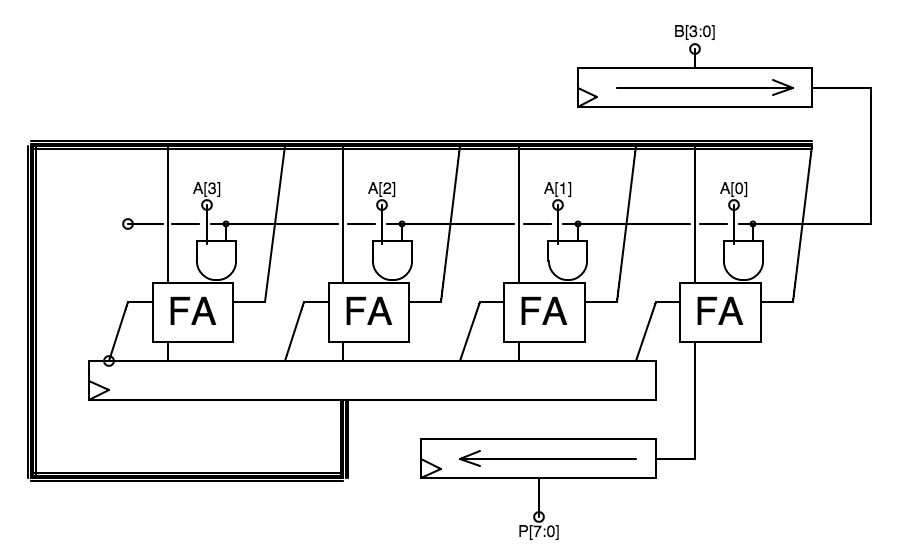4-bit Sequential Multiplier
This observation leads us to one approach to a sequential multiplier, made using a single slice which gets re-used during successive clock cycles to play the role of each of our pipeline stages. A sketch of this approach appears on the right: it uses a single pipeline stage supplying data to a pipeline register; however, most signals from this register are connected to the inputs of this single stage rather than of the next stage in the pipeline. In order to deal with the consecutive bits of the $B$ operand needed by successive pipeline stages, we load $B[3:0]$ into a shift register whose contents are shifted by one bit position at each active clock edge. Another shift register is used to collect successive bits of the product in an output register over the $2 \cdot N$ cycles it takes to do an $N$x$N$-bit multiplication.

Again, we gloss over a number of details. But such a design can be used to build an $N$-bit multiplier at a hardware cost proportional to $N$ and having a latency of $\theta(N)$ since the clock period is independent of $N$.

Multiplier Recap

#### 12.3.1.3. Multiplier Recap

The design of state-of-the-art multipliers involves a number of engineering techniques beyond our current scope; the three design examples here are chosen to illustrate tradeoffs typical of basic design decisions.

Design Cost Latency Throughput
Combinational $\theta(N^2)$ $\theta(N)$ $\theta(1/N)$
Pipelined $\theta(N^2)$ $\theta(N)$ $\theta(1)$
Sequential $\theta(N)$ $\theta(N)$ $\theta(1/N)$
Multiplier Designs
The asymptotic parameters of the three design approaches we have sketched are summarized to the left. Of course, one must be careful making design decisions based on asymptotic performance: the additive and multiplicative constants ignored by the $\theta(...)$ abstraction may dominate cost/performance decisions for particular values of $N$. Our combinational multiplier maximizes latency, but as $N$ becomes large has lower throughput than the pipelined version and is more expensive than the sequential approach.
Chapter Summary

## 12.4. Chapter Summary

This chapter explores, via simple examples, the sorts of engineering tradeoffs confronted during the design process.

Among the salient observations of this chapter:

• Power dissipation, typically proportional to the frequency of logic transitions, can be optimized by minimizing the number of transitions made during a computation.
• Minimization of combinational latency involves shortening the propagation delay along the longest input-output path. For a broad class of functions, this can be done using a tree structure, reducing the latency of an $N$-input function to $\theta(log N)$.
• Pipelining improves throughput so long as stage boundaries break long combinational paths.
• Sequential designs can exploit recurring structure replicating their function in time rather than in hardware, saving hardware cost.